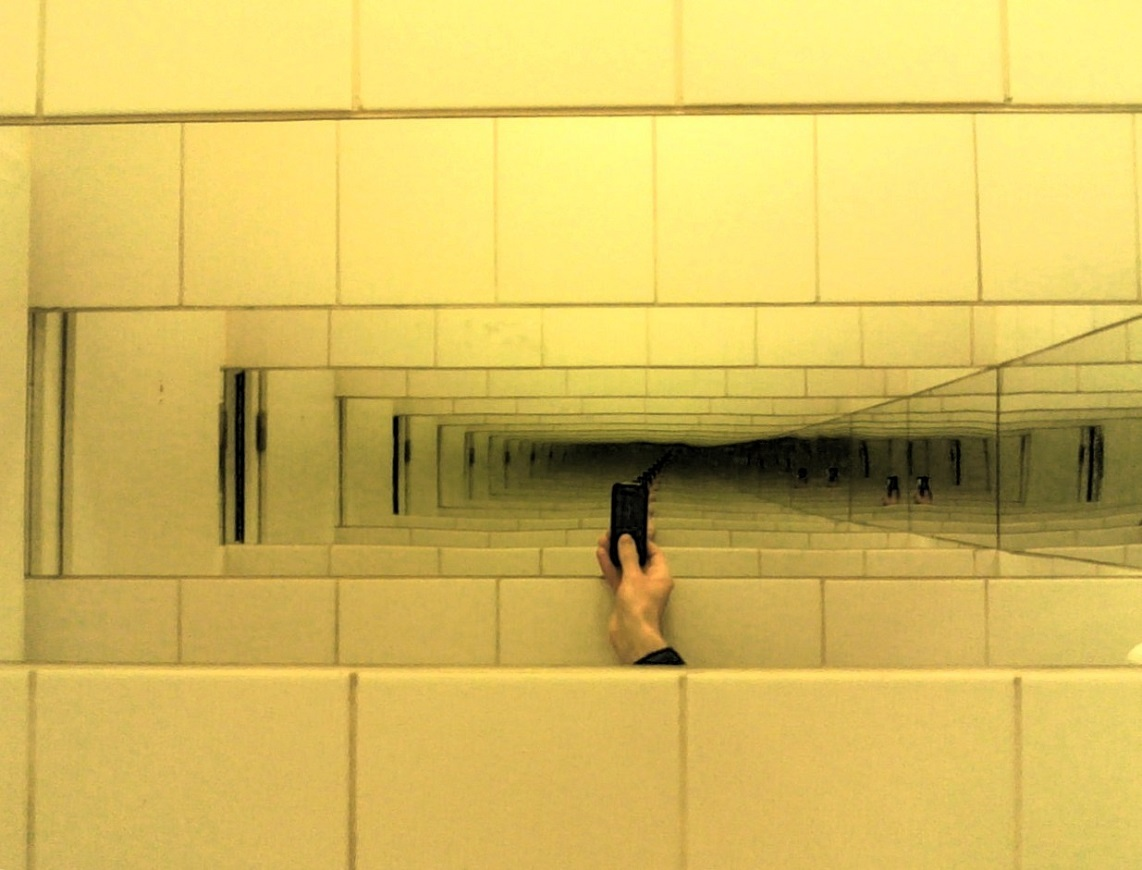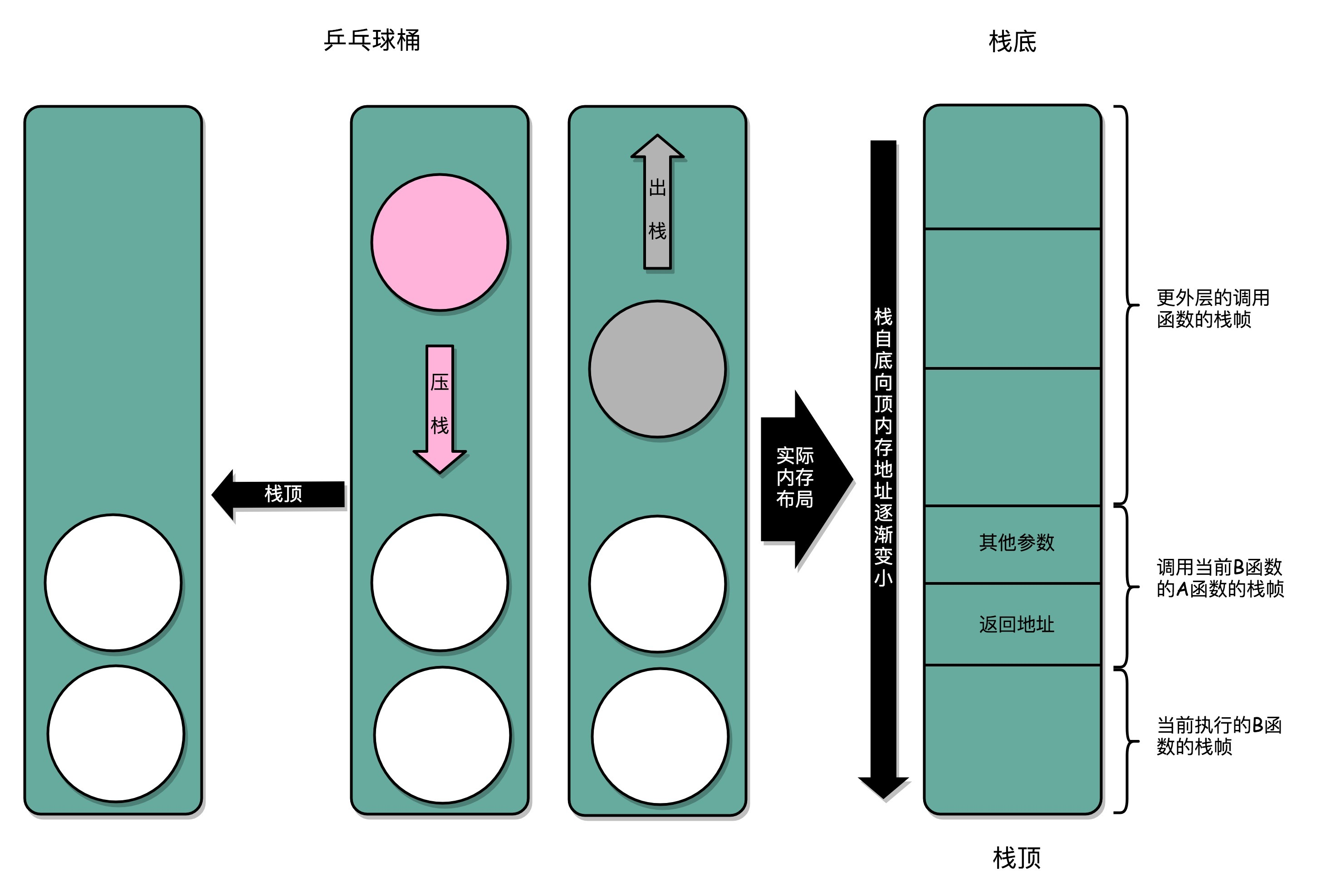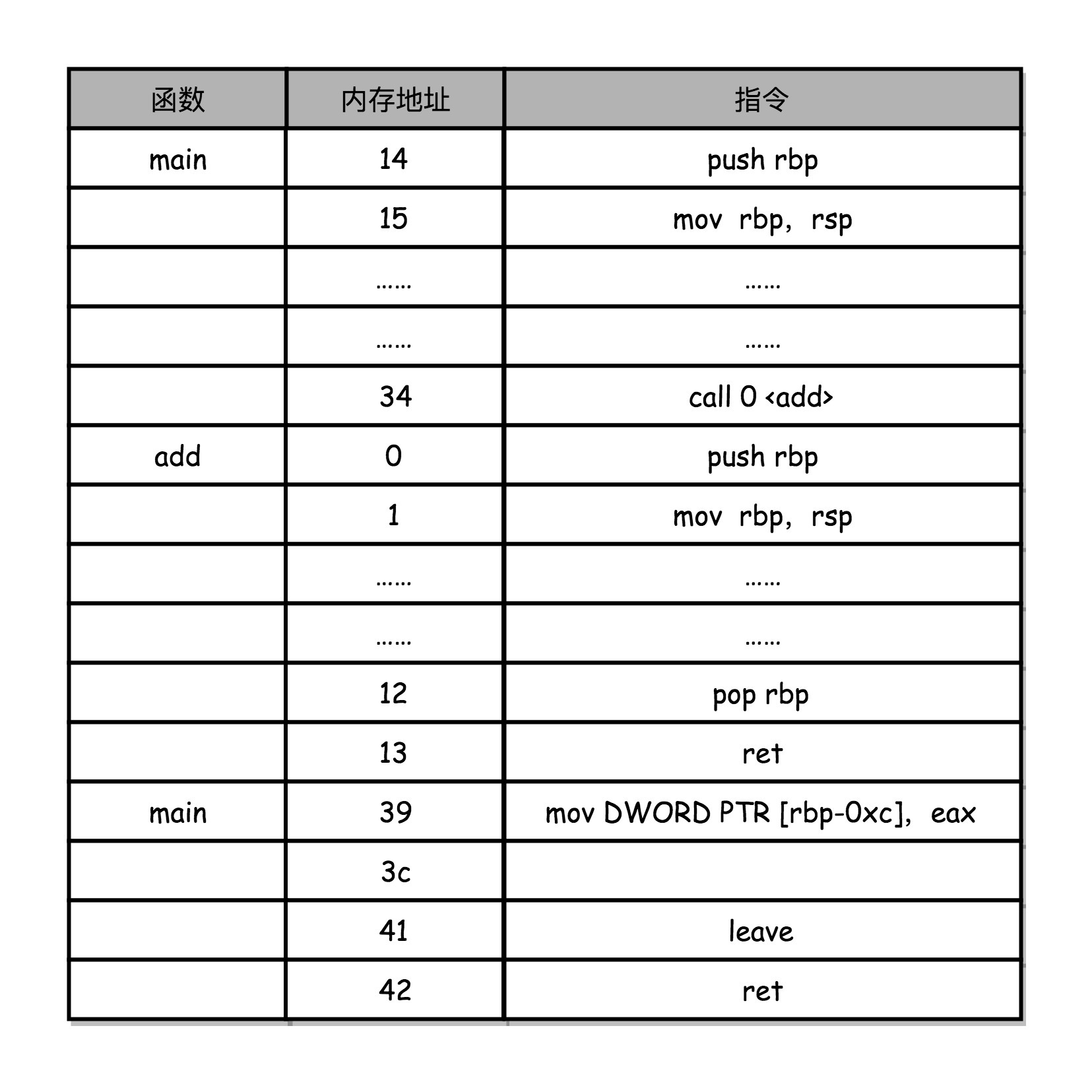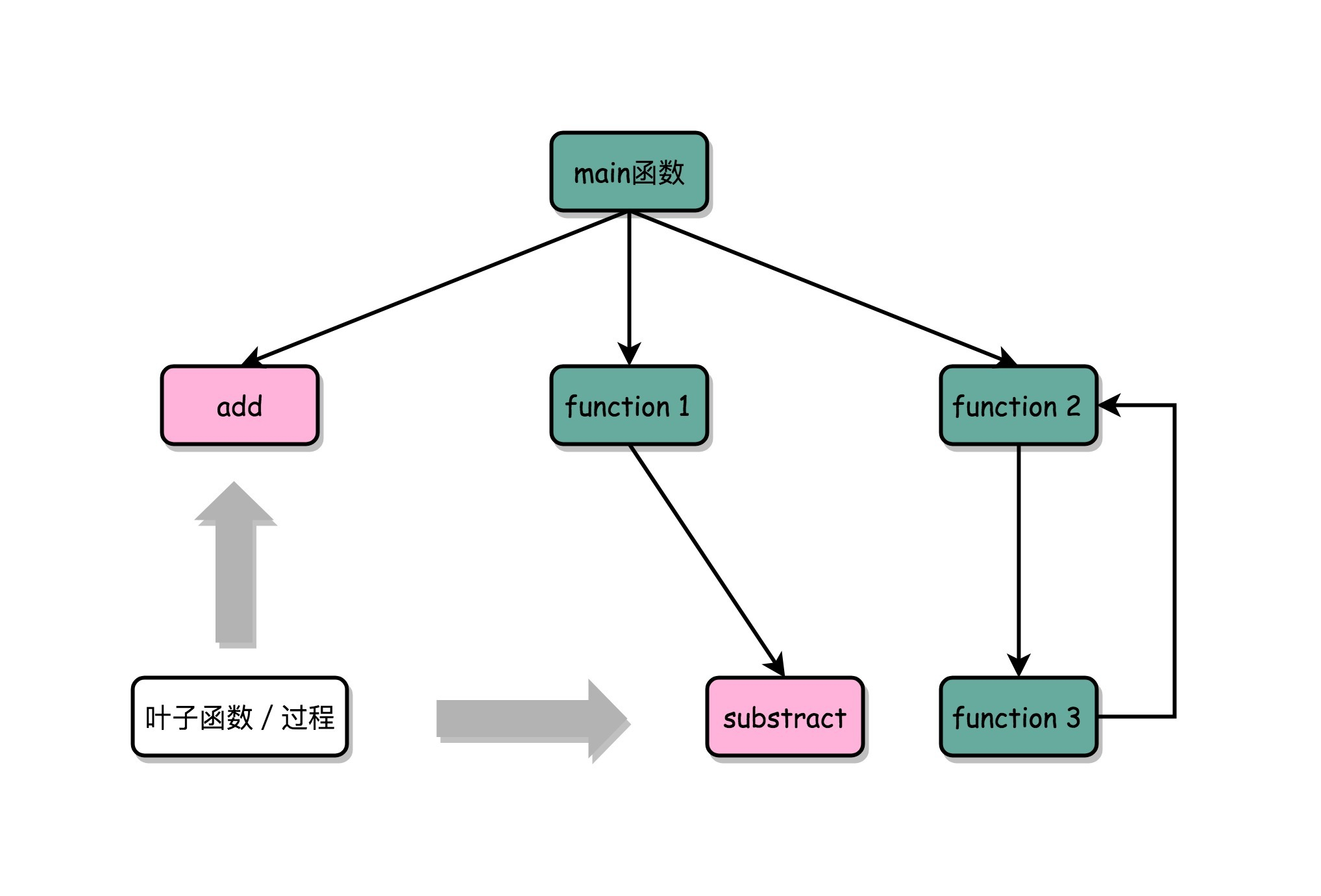07 函数调用：为什么会发生stack overflow？

## 为什么我们需要程序栈？

``````// function_example.c
#include <stdio.h>
int static add(int a, int b)
{
return a+b;
}

int main()
{
int x = 5;
int y = 10;
}
``````

``````\$ gcc -g -c function_example.c
\$ objdump -d -M intel -S function_example.o
``````

``````int static add(int a, int b)
{
0:   55                      push   rbp
1:   48 89 e5                mov    rbp,rsp
4:   89 7d fc                mov    DWORD PTR [rbp-0x4],edi
7:   89 75 f8                mov    DWORD PTR [rbp-0x8],esi
return a+b;
a:   8b 55 fc                mov    edx,DWORD PTR [rbp-0x4]
d:   8b 45 f8                mov    eax,DWORD PTR [rbp-0x8]
}
12:   5d                      pop    rbp
13:   c3                      ret
0000000000000014 <main>:
int main()
{
14:   55                      push   rbp
15:   48 89 e5                mov    rbp,rsp
18:   48 83 ec 10             sub    rsp,0x10
int x = 5;
1c:   c7 45 fc 05 00 00 00    mov    DWORD PTR [rbp-0x4],0x5
int y = 10;
23:   c7 45 f8 0a 00 00 00    mov    DWORD PTR [rbp-0x8],0xa
2a:   8b 55 f8                mov    edx,DWORD PTR [rbp-0x8]
2d:   8b 45 fc                mov    eax,DWORD PTR [rbp-0x4]
30:   89 d6                   mov    esi,edx
32:   89 c7                   mov    edi,eax
34:   e8 c7 ff ff ff          call   0 <add>
39:   89 45 f4                mov    DWORD PTR [rbp-0xc],eax
3c:   b8 00 00 00 00          mov    eax,0x0
}
41:   c9                      leave
42:   c3                      ret
``````Infinite Mirror Effect，如果函数 A 调用 B，B 再调用 A，那么代码会无限展开，图片来源## 如何构造一个 stack overflow？

``````int a()
{
return a();
}

int main()
{
a();
return 0;
}
``````

## 如何利用函数内联进行性能优化？

``````#include <stdio.h>
#include <time.h>
#include <stdlib.h>

int static add(int a, int b)
{
return a+b;
}

int main()
{
srand(time(NULL));
int x = rand() % 5
int y = rand() % 10;
printf("u = %d\n", u)
}
``````

``````\$ gcc -g -c -O function_example_inline.c
\$ objdump -d -M intel -S function_example_inline.o
``````

``````    return a+b;
``````